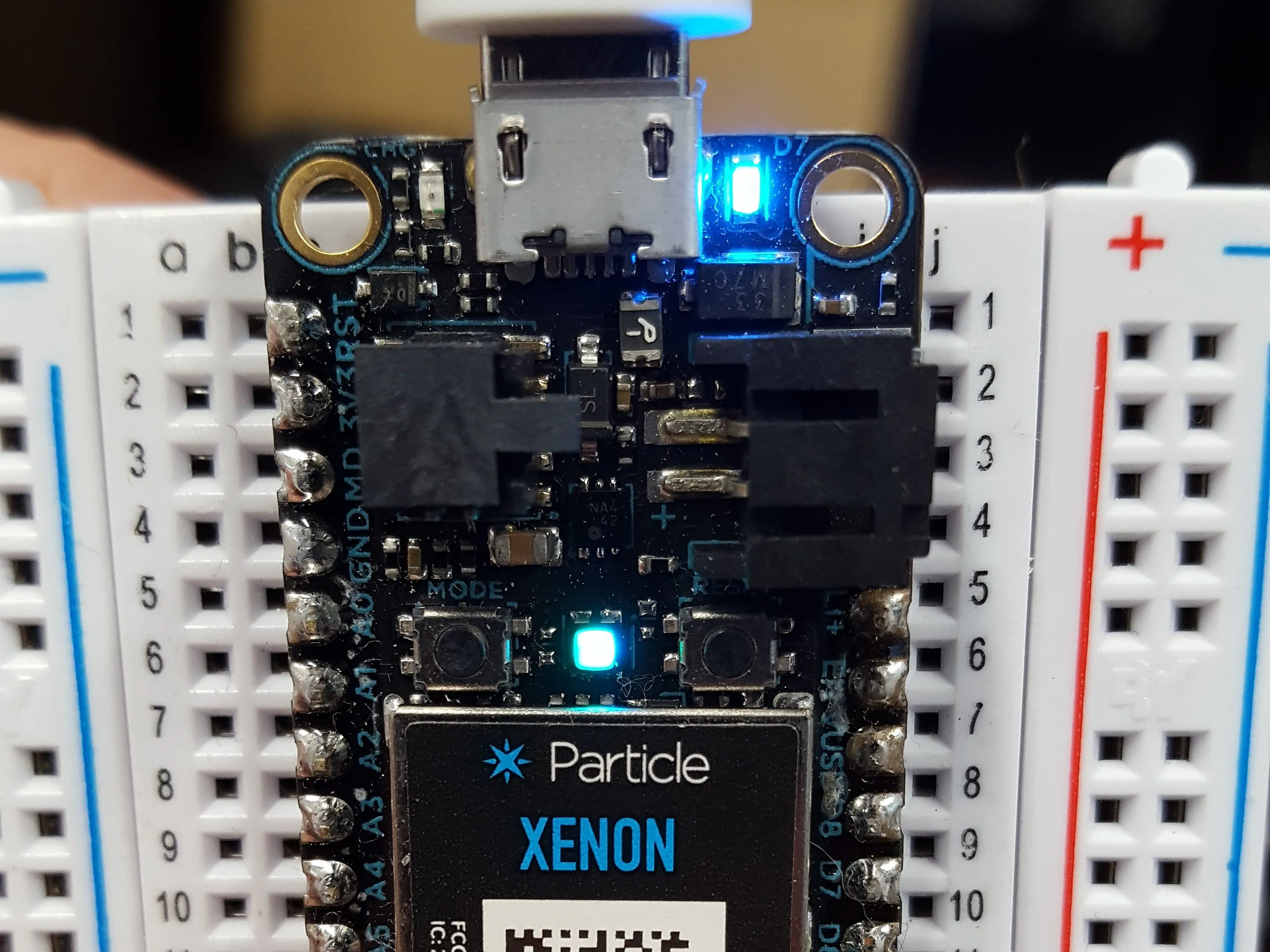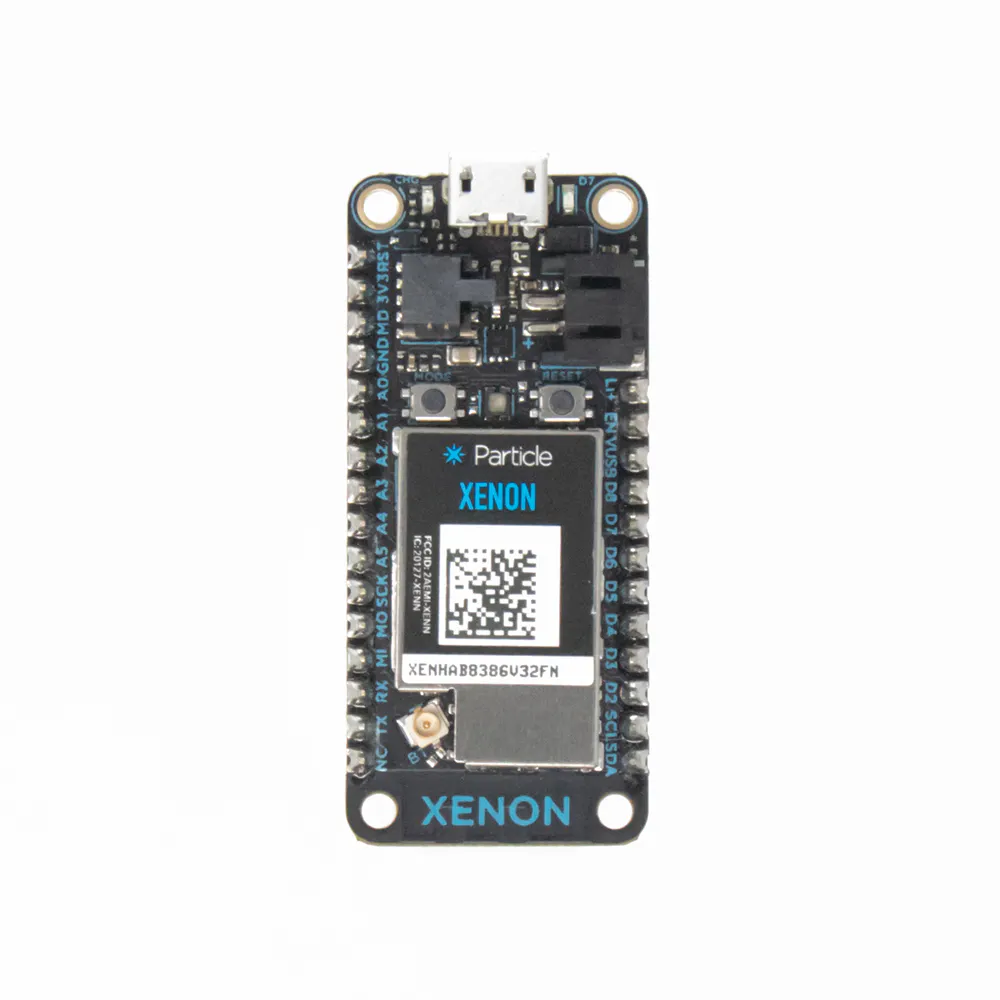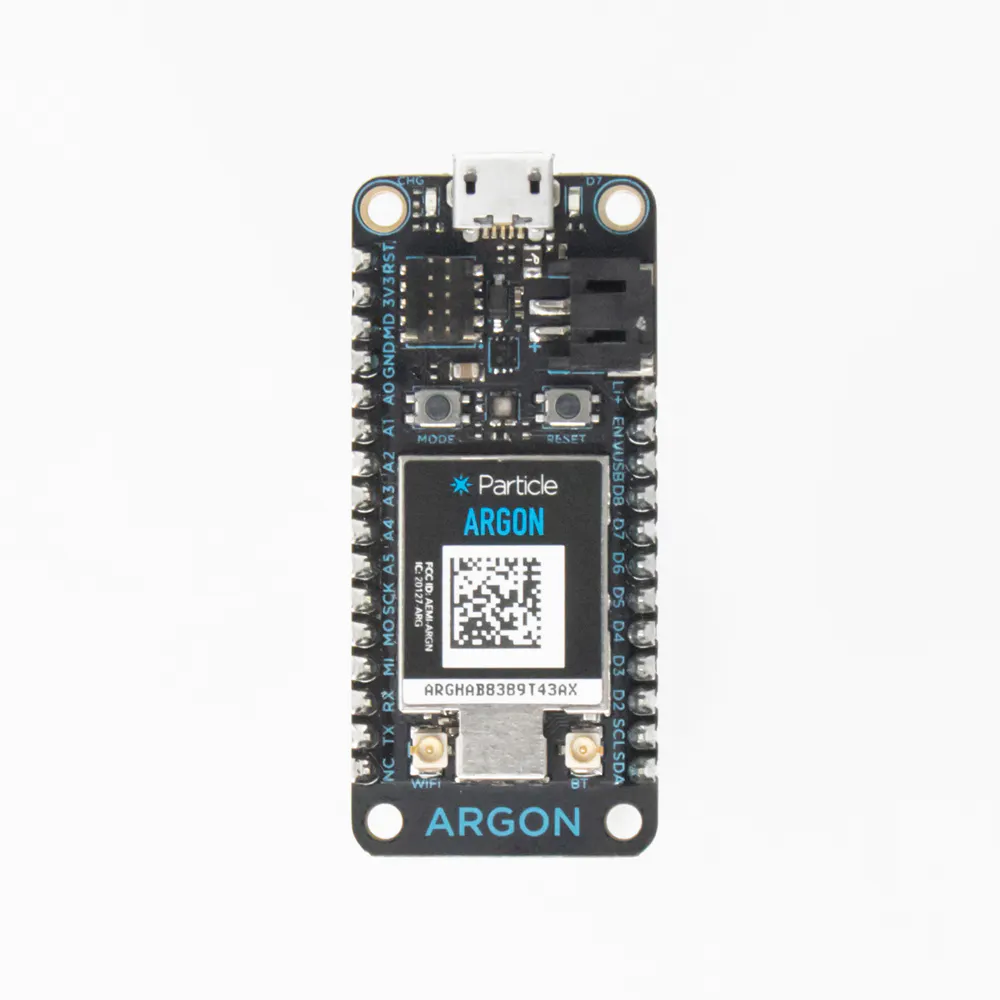# Particle Mesh Pi Day 2019

My entry for the Particle.io community Pi Day contest 2019. I used a Particle Xenon to flash 100 decimal places of Pi in Morse code.

BeginnerFull instructions provided1 hour666## Things used in this project

### Hardware componentsParticle Xenon Does the actual LED blinking of Morse Code.
×1Particle Argon Acts as a gateway to send the compiled code to the Xenon endpoint over-the-air (OTA).
×1

## Schematics

Watch 50 seconds of the number Pi being flashed in Morse code.

## Code

### Pi-day Morse Code

C/C++
You can flash this to just about any Particle device (Photon, Electron, Argon, Xenon, Boron).
```.
#include <math.h>

//Original morse_code by LED
/*
This code was written by Ebrahim Bawazir in 2013 and updated in 2015
U R FREE TO USE IT AND MODIFY IT AS U WANT
*****************
*/

//Adapted for use to flash 100 digits of Pi by ninjatill
//for the Particle.io community Pi-day contest

int LED = D7;//the led pin
char piArray[] = "3.141592653589793238462643383279502884197169399375105820974944592307816406286208998628034825342117067";
char input;
uint8_t charIndex = 0;
unsigned long startMillis = 0;
unsigned long delayDuration = 10000;

float speedMultiplier = 2;

enum states:int {
PROCESS
, DELAY
};

states myState;
states lastState;

void setup () {
pinMode(LED,OUTPUT);//tell that the 13 pin is an output
digitalWrite(LED, HIGH);
delay(500);
digitalWrite(LED, LOW);
myState = states::DELAY;
lastState = states::PROCESS;
}

void loop () {
if (myState != lastState) {
lastState = myState;

switch (myState) {
case PROCESS:
charIndex = 0;
break;
case DELAY:
startMillis = millis();
break;
}
}

switch (myState) {
case PROCESS: // code to be executed if n = 1;
ProcessArray();
break;
case DELAY: // code to be executed if n = 2;
if (millis() - startMillis > delayDuration) {
myState = PROCESS;
}
break;
}

}

void ProcessArray() {
if (charIndex < arraySize(piArray)) {
input = piArray[charIndex];
if (input == 'a' || input == 'A') {lA();}//if the input is a or A go to function lA
if (input == 'b' || input == 'B') {lB();}//same but with b letter
if (input == 'c' || input == 'C') {lC();}
if (input == 'd' || input == 'D') {lD();}
if (input == 'e' || input == 'E') {lE();}
if (input == 'f' || input == 'F') {lF();}
if (input == 'g' || input == 'G') {lG();}
if (input == 'h' || input == 'H') {lH();}
if (input == 'i' || input == 'I') {lI();}
if (input == 'j' || input == 'J') {lJ();}
if (input == 'k' || input == 'K') {lK();}
if (input == 'l' || input == 'L') {lL();}
if (input == 'm' || input == 'M') {lM();}
if (input == 'n' || input == 'N') {lN();}
if (input == 'o' || input == 'O') {lO();}
if (input == 'p' || input == 'P') {lP();}
if (input == 'q' || input == 'Q') {lQ();}
if (input == 'r' || input == 'R') {lR();}
if (input == 's' || input == 'S') {lS();}
if (input == 't' || input == 'T') {lT();}
if (input == 'u' || input == 'U') {lU();}
if (input == 'v' || input == 'V') {lV();}
if (input == 'w' || input == 'W') {lW();}
if (input == 'x' || input == 'X') {lX();}
if (input == 'y' || input == 'Y') {lY();}
if (input == 'z' || input == 'Z') {lZ();}
if (input == '1') {n1();}// the numbers
if (input == '2') {n2();}
if (input == '3') {n3();}
if (input == '4') {n4();}
if (input == '5') {n5();}
if (input == '6') {n6();}
if (input == '7') {n7();}
if (input == '8') {n8();}
if (input == '9') {n9();}
if (input == '0') {n0();}
if (input == '.') {space();}//the space
if (input == ' ') {space();}//the space
charIndex++;
} else {
myState = states::DELAY;
}
}

//f=unctions for the letters and the numbers
void lA () {dot();dash();shortspace();}//letter A in morse code!
void lB () {dash();dot();dot();dot();shortspace();}//same for B
void lC () {dash();dot();dash();dot();shortspace();}
void lD () {dash();dot();dot();shortspace();}
void lE () {dot();shortspace();}
void lF () {dot();dot();dash();dot();shortspace();}
void lG () {dash();dash();dot();shortspace();}
void lH () {dot();dot();dot();dot();shortspace();}
void lI () {dot();dot();shortspace();}
void lJ () {dot();dash();dash();dash();shortspace();}
void lK () {dash();dot();dash();shortspace();}
void lL () {dot();dash();dot();dot();shortspace();}
void lM () {dash();dash();shortspace();}
void lN () {dash();dot();shortspace();}
void lO () {dash();dash();dash();shortspace();}
void lP () {dot();dash();dash();dot();shortspace();}
void lQ () {dash();dash();dot();dash();shortspace();}
void lR () {dot();dash();dot();shortspace();}
void lS () {dot();dot();dot();shortspace();}
void lT () {dash();shortspace();}
void lU () {dot();dot();dash();shortspace();}
void lV () {dot();dot();dot();dash();shortspace();}
void lW () {dot();dash();dash();shortspace();}
void lX () {dash();dot();dot();dash();shortspace();}
void lY () {dash();dot();dash();dash();shortspace();}
void lZ () {dash();dash();dot();dot();shortspace();}
void n1 () {dot();dash();dash();dash();dash();shortspace();}//number 1 in morse code
void n2 () {dot();dot();dash();dash();dash();shortspace();}
void n3 () {dot();dot();dot();dash();dash();shortspace();}
void n4 () {dot();dot();dot();dot();dash();shortspace();}
void n5 () {dot();dot();dot();dot();dot();shortspace();}
void n6 () {dash();dot();dot();dot();dot();shortspace();}
void n7 () {dash();dash();dot();dot();dot();shortspace();}
void n8 () {dash();dash();dash();dot();dot();shortspace();}
void n9 () {dash();dash();dash();dash();dot();shortspace();}
void n0 () {dash();dash();dash();dash();dash();shortspace();}
void period () {dot();dash();dot();dash();dot();dash();shortspace();}
void space () {delay (ceil((float)1200 / speedMultiplier));}//space between words
void dot () {digitalWrite(LED,HIGH); delay (ceil((float)300 / speedMultiplier)); digitalWrite(LED,LOW); delay (ceil((float)300 / speedMultiplier));}//the dot this code make the led on for 300 than off for 300
void dash () {digitalWrite(LED,HIGH); delay (ceil((float)900 / speedMultiplier)); digitalWrite(LED,LOW); delay (ceil((float)300 / speedMultiplier));}//the dash this code make the led on for 900 than off for 300
void shortspace () {delay(ceil((float)600 / speedMultiplier));}//space between letters
/*done*/
```

## Credits

### Tim Jarosz

1 project • 1 follower
Hobbyist engineer. Mainly using Particle Photon (and some Arduino Unos) for IoT.
Thanks to Ebrahim Bawazir.